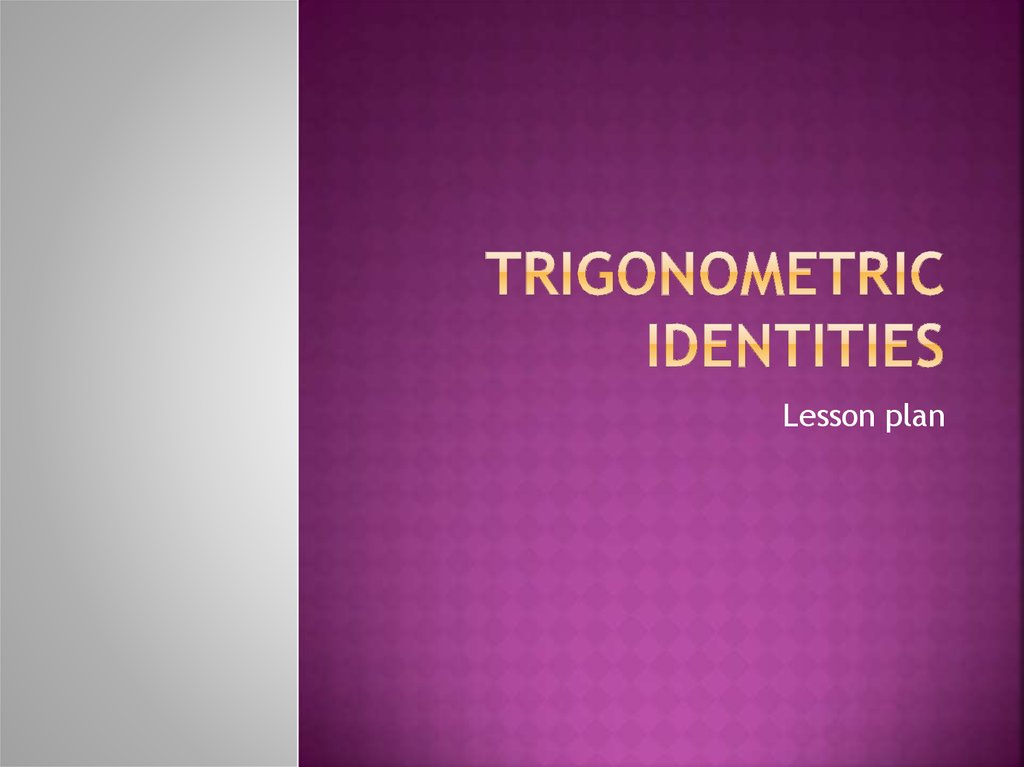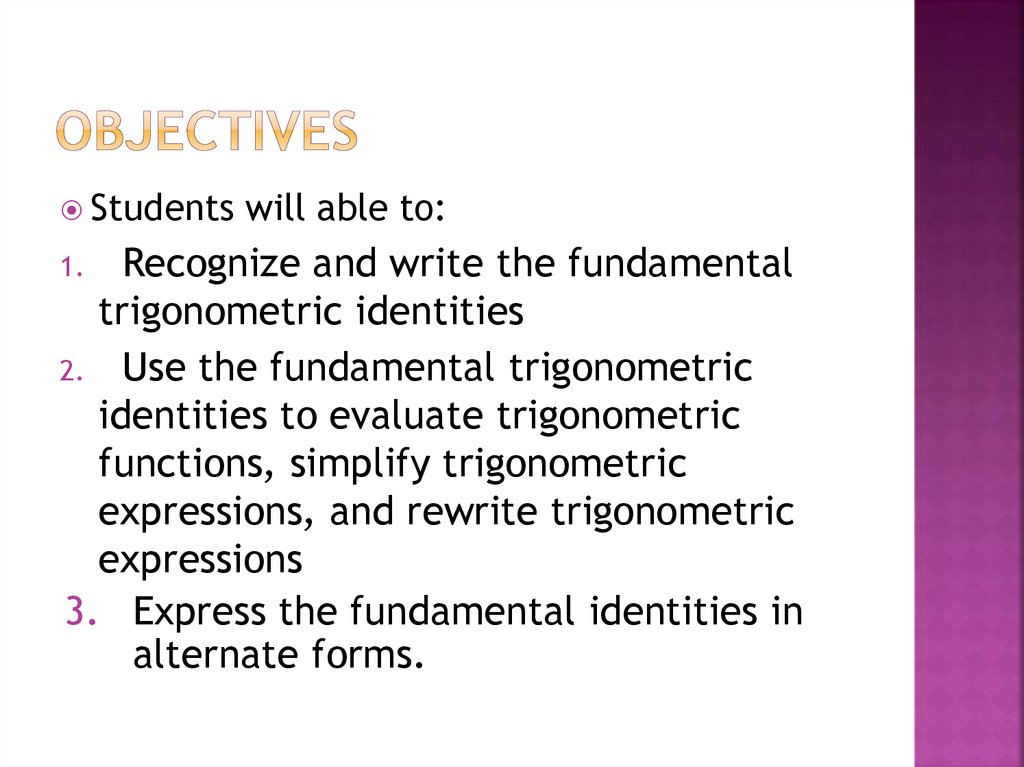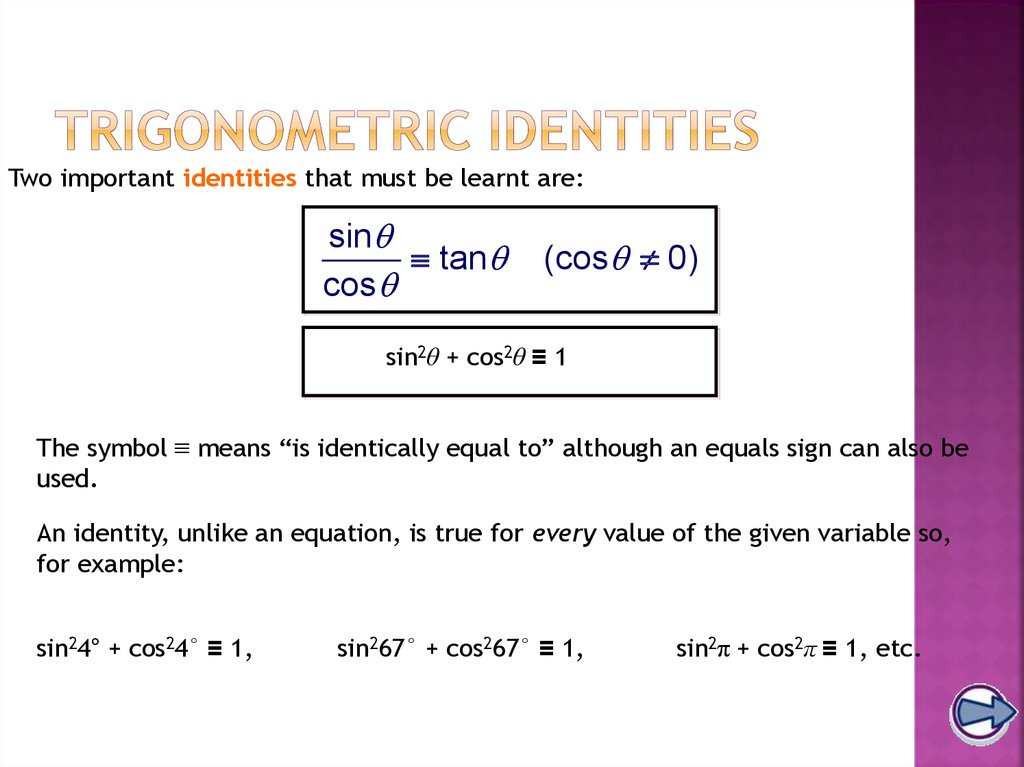# Trigonometric identities

Lesson plan

## 2. Objectives

Students
will able to:
Recognize and write the fundamental
trigonometric identities
2. Use the fundamental trigonometric
identities to evaluate trigonometric
functions, simplify trigonometric
expressions, and rewrite trigonometric
expressions
3. Express the fundamental identities in
alternate forms.
1.

## 3. Trigonometric identities

Two important identities that must be learnt are:
sin
tan
cos
(cos 0)
sin2θ + cos2θ ≡ 1
The symbol ≡ means “is identically equal to” although an equals sign can also be
used.
An identity, unlike an equation, is true for every value of the given variable so,
for example:
sin24° + cos24° ≡ 1,
sin267° + cos267° ≡ 1,
sin2π + cos2π ≡ 1, etc.

## 4. Trigonometric identities

We can prove these identities by considering a right-angled triangle:
r
y
θ
x
y
and cos =
sin =
r
r
y
sin
y
= r = = tan as required.
x
cos
x
r
x
Also:
2
y x
2
2
sin + cos = +
r r
2
x2 + y 2
=
r2
But by Pythagoras’ theorem x2 + y2 = r2 so:
2
r
sin2 + cos2 = 2 = 1 as required.
r

## 5. Fundamental Trigonometric Identities

Pythagorean Identities
sin 2 u cos 2 u 1
1 tan 2 u sec 2 u
1 cot u csc u
2
2
Even/Odd Identities
sin( u) sin u
cos( u) cosu
tan( u) tan u
csc( u) csc u
sec( u) sec u
cot( u) cot(u)

## 6. Fundamental Trigonometric Identities

Cofunction Identities
sin u = cos u
2
tan u cot u
2
sec u csc u
2
cos u sin u
2
cot u tan u
2
csc u sec u
2

## 7. 5.5 & 5.6 Double & Half Angle and Power Reducing Formulas

Double-Angle
sin 2 = 2 sin cos
cos 2 = cos2 - sin2
= 2 cos2 - 1
= 1 – 2 sin2
Power Reducing Formulas
sin2 = 1 – cos 2
2
cos2 = 1 + cos 2
2
tan 2 = 2 tan
1 – tan2
Half Angle
sin =
2
1 – cos
2
tan =
tan2 = 1 – cos 2
1 + cos 2
cos =
1 – cos
=
2
sin
1 + cos
2
sin
2
1-cosA
= +/1+cosA
1 + cos

## 8. Product-to-Sum & Sum to Product Formulas

Product to Sum
sin sin = ½ [cos ( - ) – cos ( + )]
cos cos = ½ [cos ( - ) + cos ( +
)]
sin cos = ½ [sin ( + ) + sin ( - )]
cos sin = ½ [cos ( + ) – sin ( - )]
Sum to Product
sin + sin = 2 sin + cos -
2
2
sin - sin = 2 sin - cos +
2
2
cos + cos = 2 cos + cos -
2
2
cos - cos = -2 sin + sin -
2
2

## 9.

Example: If
and Ө is in quadrant II,
find each function value. (Cont.)
b) sin Ө
Tip: Use Quotient Identities.
c) cot ( Ө )
Tip: Use Reciprocal and
Negative-Angle Identities.
9

## 10. Simplifying a Trigonometric Expression

sin x cos x sin x
2
2
2
sec x(1 sin x)
2
tan x
sec 2 x

## 11. 5.2 Verifying Identities

Identity – An equation that is satisfied for all meaningful replacements of the
Verifying Identities – Show/Prove one side of an equation actually equals th
Example: Verify the identity:
Techniques for Verifying Identitie
1. Change to Sine and Cosine
cot + 1 = csc (cos + sin )
= 1/sin (cos + sin )
2.
= (1/sin ) cos + (1/sin ) sin
= cos / sin + sin / sin
3.
= cot + 1
4.
We will do other examples in class
Use Algebraic Skills – factoring
Use Pythagorean Identities
Work each side separately
(Do NOT add to both sides, etc
5. For 1 – sin x, try multiplying
numerator & denominator by
1 + sin x to obtain 1 = sin2 x